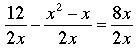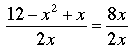SEARCH HOMEMath Central Quandaries & QueriesQuestion from leah, a student: If 6/x - (x-1)/2 = 4 then the LCD is 2x, right? so you get 12/2x - (x2-x)/2x = 8x/2x, right? then what do you do with the 2x when you turn it into a quadratic equation?Hi Leah,

You're good so far. You have.

Since thw two fractions on the left have the same denominator you can combine them to get.

At this point you have two equal fractions with the equal denominators and hence they have equal numerators, that is

12 - x2 + x = 8x.

This is the quadratic you need to solve.

There is one warning you need to be aware of. The original equation, 6/x - (x-1)/2 = 4, has a zero in the denominator of one of the fractions so the equation is not valid when x = 0. The quadratic you are to solve might very well have x = 0 as a solution and thus lead you to believe that x = 0 is a solution of the original problem, which it is not. This is precisely why you need to check that the solutions to the quadratic are actually solutions to the original problem.

PennyMath Central is supported by the University of Regina and The Pacific Institute for the Mathematical Sciences.